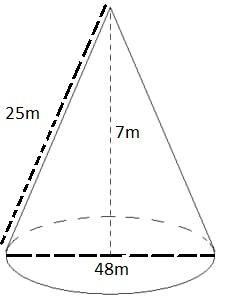Courses
Courses for Kids
Free study material
Free LIVE classes
More# The heap of wheat is in the form of a cone whose diameter is $48m$ and height is $7m$. Find the volume of the heap. If it is covered by canvas to protect it from rain. Find the cost of the canvas required. Given that the rate of canvas is $Rs.7\,per\,100c{m^2}$.

Last updated date: 24th Mar 2023
Total views: 206.4k
Views today: 3.83kVerified
206.4k+ views
Hint: The area can be defined as the space occupied by a flat surface of an object. The area is the number of unit squares closed by figure. Perimeter is the total length of the sides of the two dimensional shape. Perimeter is always less than the area of the given figure. Because the perimeter is outer and the area is inner property. Volume is the capacity which hold by objects
As we know that
$\therefore V = \dfrac{1}{3}\pi {r^2}h$
Here
V=volume
h=height

Complete step-by-step solution:Given,
Diameter of heap,$d = 48m$
Radius of heap, $r = \dfrac{{48}}{2}m$
Radius of heap, $r = 24m$
Height of heap, $h = 7m$
Rate of canvass $Rs.7\,per\,100c{m^2}$
Volume=?
Slant height of heap, $l = ?$
As we know that
$\therefore l = \sqrt {{h^2} + {r^2}}$
Put the value
$\Rightarrow l = \sqrt {{7^2} + {{24}^2}}$
$\Rightarrow l = \sqrt {49 + 576}$
$\Rightarrow l = \sqrt {625}$
$\Rightarrow l = 25m$
Now the volume of heap
As we know that
$\therefore V = \dfrac{1}{3}\pi {r^2}h$
Put the value
$\Rightarrow V = \dfrac{1}{3} \times \dfrac{{22}}{7} \times {(24)^2} \times 7$
Simplify
$\Rightarrow V = 22 \times 24 \times 8$
$\Rightarrow V = 4224{m^3}$
Now area covered by canvas
As we know that
$\therefore A = \pi rl$
Put the value
$\Rightarrow A = \dfrac{{22}}{7} \times 24 \times 25$
Simplify
$\Rightarrow A = \dfrac{{13200}}{7}$
$\Rightarrow A = 1885.71{m^2}$
Now
The price of canvas,
$\Rightarrow \text{Price of canvas} = \text{Area covered} \times \text{Rate of canvas}$
Put the value
$\Rightarrow \text{Price of canvas} = 1885.71 \times \dfrac{7}{{100}}$
$1{m^2} = 10000c{m^2}$
$\Rightarrow \text{Price of canvas} = 1885.71 \times \dfrac{7}{{100}} \times 10000$
$\Rightarrow \text{Price of canvas} = Rs.1320000$

Note: The slant height of an object (such as frustum, cone, and pyramid) is the distance measured along a lateral face from the base to the apex along the “center” of the face. As we know that the slant height is given by
$\therefore l = \sqrt {{h^2} + {r^2}}$
Here
h=height of cone